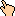## 1996-10 Solution

Clearly one simple way to prove the set is infinite is to find a generator number or pattern that allows someone to generate an infinite series of recombinant numbers. Bar that, here is a strong argument to argue that the set is infinite:

Take two simple recombinant numbers:

25 = 52
216 (= 63) = 6(1+2)

The numbers 5 and 6 are unique in that every number that's a power of these contains that digit in the one's place. So you can recombine the number by using all the digits to the left of the ones place to create the exponent in the equation: 5(blah) and 6(blah). Now the 'blah' numbers grow slowly, but the number of digits grows almost as fast (about half as fast on average). So, for example, to create 664, which is:

63340286662973277706162286946811886609896461828096

we have 49 digits to combine together somehow to get 64. Very very very easy - the simplest way is to pick two 8s to make the 64 and add that to the product of the rest of the numbers (there's a 0 in there, so that goes away). In general if I gave you x numbers to recombine together to make a number at most twice x, it would be trivial when x gets large as is this case. If the power we choose is itself a power of 5 or 6, this means that we can write the number as 5(5blah) which means if there is a 5 somewhere else in the sequence (aside from the one's place) that we remove the 5, use which reduces the number of digits we have to use (x) by only ONE, but it reduces the number we have to combine to by a factor of 5. We continue this by choosing numbers which are of the form 5(5(5...etc)) e.g.
55 = 3125 = 5(3+2)*1
5(55) =

```191101259794547752035640455970396459919808104899009433713951278924652\
053024261580301205938651973985026558644015579446223535921278867380697\
228841014691598660208796189675719570183928166033804761122597553362610\
100148265112341314776825241149309444717696528275628519673751439535754\
247909321920664188301178716912255242107005070906467438287085144995025\
658619446154318351137984913369177992812743384043154923685552678359637\
410210533154603135372532574863690915977869032826645918298381523028693\
657287369142264813129174376213632573032164528297948686257624536221801\
767322494056764281936007872071383707235530544635615394640118534849379\
271951459450550823274922160584891291094518995994868619954314766693801\
303717616359259447974616422005088507946980448713320513316073913423054\
019887257003832980124605019701346739717590902738949392381731578699684\
589979478106804282243609378394633526542281570430283244238551508231649\
096728571217170812323279048181726832751011274678231741098588868370852\
200071173349225391332230075614718042900752767779335230620061828601245\
525424306100689480544658470482065098266431936096038873625851074707434\
063628697657670269925864995355797631817390255089133122329474393034395\
616132833407283166349825814522686200430779908468810380418736832480090\
387359621291963360258312078167367374253332287929690720549059562140688\
882599124458184237959786347648431567376092362509037151179894142426227\
022006628648686786871018298087280256069310194928083082504419842479679\
205890881711232719230145558291674679519743054802640464685400273399386\
079859446596150175258696581144756851004156868773090371248253534383928\
539759874945849705003822501248928400182659005625128618762993804440734\
014234706205578530532503491818958970719930566218851296318750174353596\
028220103821161604854512103931331225633226076643623668829685020883949\
614283048473911399166962264994856368523471287329479668088450940589395\
110465094413790950227654565313301867063352132302846051943438139981056\
140065259530073179077271106578349417464268472095613464732774858423827\
489966875505250439421823219135722305406671537337424854364566378204570\
165459321815405354839361425066449858540330746646854189014813434771465\
0315037954175778622811776585876941680908203125
```

and all we have to do is pull *2* 5s out of this mess and a 0 to make the others go away.

WWW Maven: Dan Garcia (ddgarcia@cs.berkeley.edu)Send me feedback